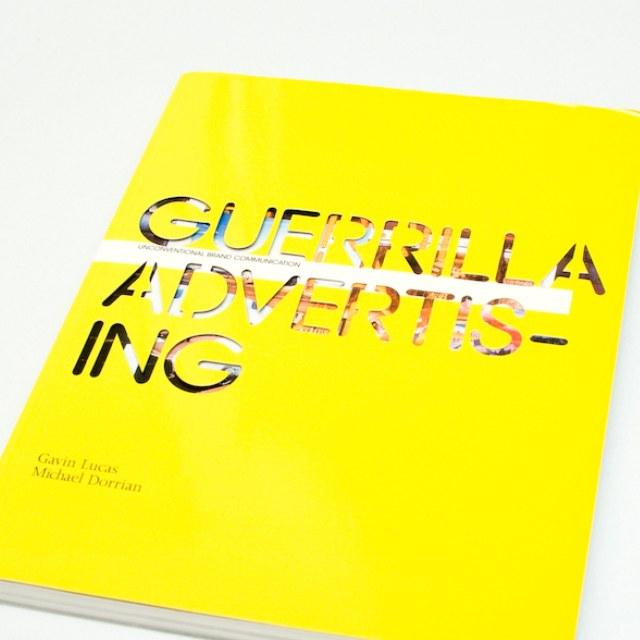Linus Bohman / CC BY

## 我们看一眼广告值多少钱？创造了多少价值？连续创业者/宽客

1.电商搜索页面
，淘宝为例：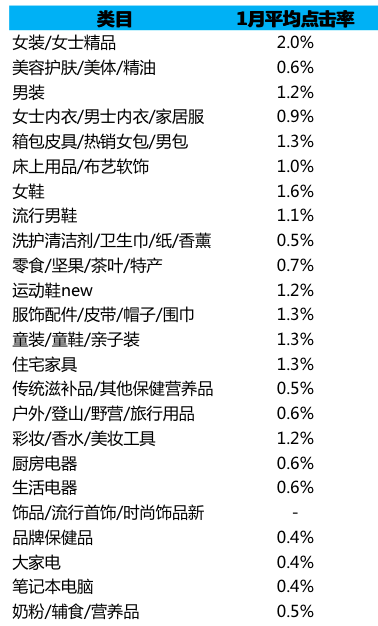CTR（点击率）在 2%，即百次展现点击 2 次

``````CTR*CPC*17+CPM*0.001*3
``````

``````0.02*3*17+10*0.001*3=1.05
``````

2.电商首页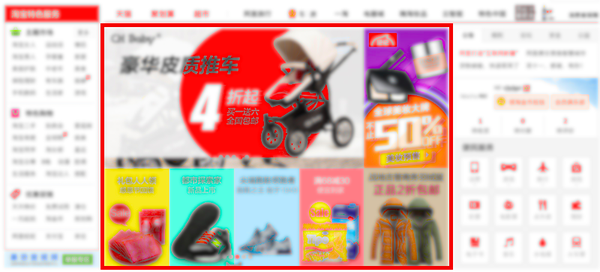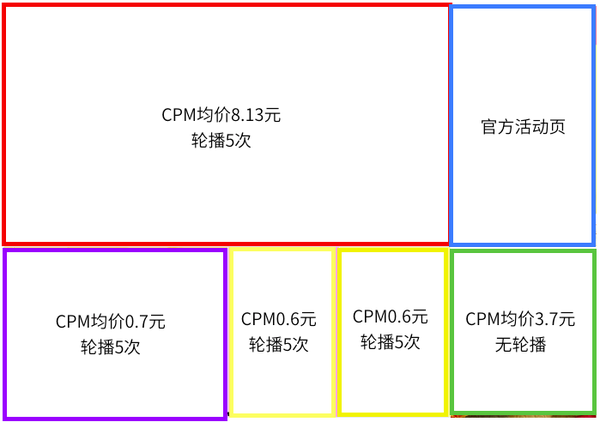``````((CPM1+CPM2+CPM3+CPM4)*5+CPM5)*0.001/0.8
``````

``````((8.13+0.7+0.6+0.6)*5+3.7)*0.001/0.8=0.0673125
``````

3.视频：优酷为例``````CPM*4*0.001/0.8=0.15
``````

4.百度搜索页面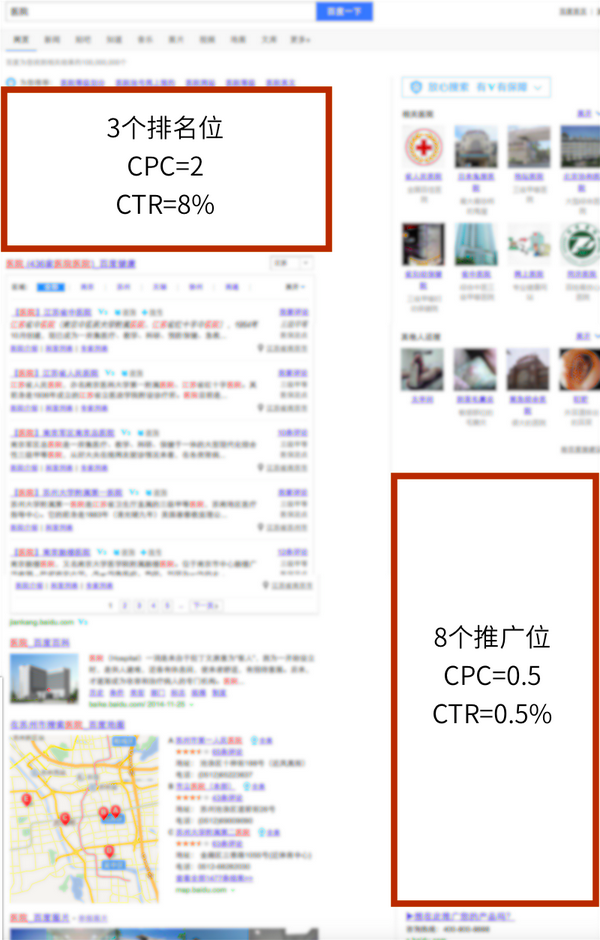``````CTR1*CPC1+CTR*CPC2
``````

``````0.08*2+0.005*0.5=0.1625
``````

5.QQ 聊天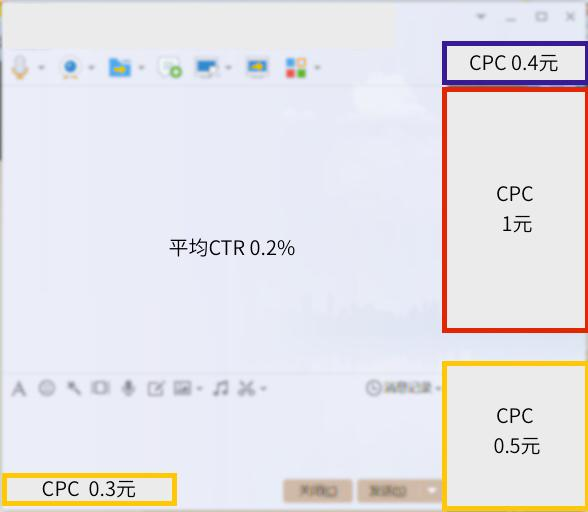``````(CPC1+CPC2+CPC3+CPC4)*CTR
``````

``````(0.3+0.5+1+0.4)*0.002=0.0044
``````

6.知乎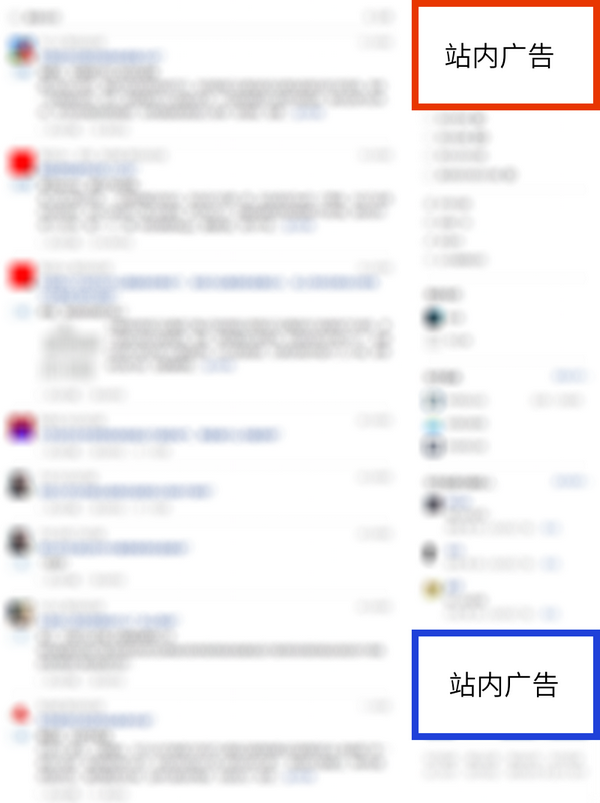7.移动端：淘宝 App``````CPM*0.001*3/0.8
``````

8.微信、手机 QQ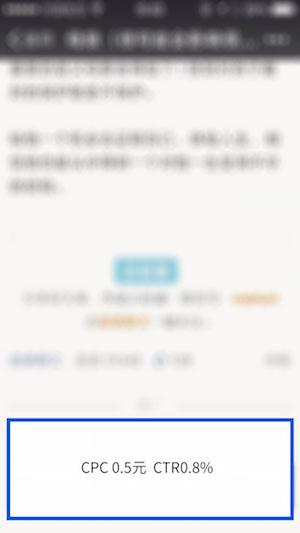``````CPC*CTR
``````